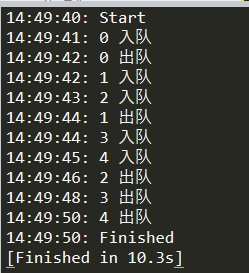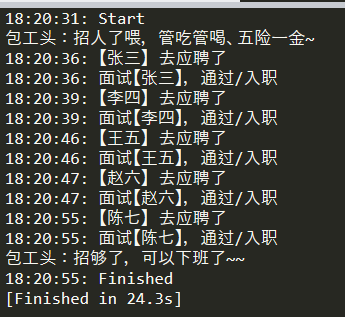# python 队列(queue)阻塞

import queue

def 入队阻塞():
q = queue.Queue(maxsize=3)
for i in range(4):
q.put('任务' + str(i+1))

print('Finished')

if __name__ == '__main__':
入队阻塞()

import queue

def 入队阻塞():
q = queue.Queue(maxsize=3)
for i in range(4):
try:
q.put('任务' + str(i+1), block=True, timeout=3)
except queue.Full:
print('任务%d: 队列已满，写入失败' % (i+1))

print('Finished')

if __name__ == '__main__':
入队阻塞()

import queue

def 出队阻塞():
q = queue.Queue(maxsize=3)
for i in range(3):
try:
q.put_nowait('任务' + str(i+1))
except queue.Full:
print('full')

for i in range(4):

print('Finished')

if __name__ == '__main__':
出队阻塞()

import queue

def 出队阻塞():
q = queue.Queue(maxsize=3)
for i in range(3):
try:
q.put_nowait('任务' + str(i+1))
except queue.Full:
print('full')

for i in range(4):
try:
except queue.Empty:
print('队列为空，get失败')

print('Finished')

if __name__ == '__main__':
出队阻塞()

import queue

def 消费阻塞():
q = queue.Queue(maxsize=3)
for i in range(3):
try:
q.put_nowait('任务' + str(i+1))
except queue.Full:
print('full')

for i in range(2):
try:
except queue.Empty:
print('队列为空，get失败')
# 阻塞队列
q.join()
print('Finished')

if __name__ == '__main__':
消费阻塞()

import time
import queue
import asyncio

def get_now():
return time.strftime('%X')

# 入队
async def qput(q):
for i in range(5):
# 每1秒写入一个元素
await asyncio.sleep(1)
try:
await q.put(i)
print('%s: %d 入队' % (get_now(), i))
except queue.Full:
print('Full')

# 出队
async def qget(q):
for i in range(5):
# 每2秒消费一个元素
await asyncio.sleep(2)
try:
item = await q.get()
print('%s: %d 出队' % (get_now(), item))
except queue.Empty:
print('Empty')

async def main():
q = asyncio.Queue(maxsize=3)

print('%s: Start' % get_now())
await asyncio.gather(qput(q), qget(q))
print('%s: Finished' % get_now())

if __name__ == '__main__':
asyncio.run(main())六、出队阻塞的作用

import time
import queue
import asyncio
import random

def get_now():
return time.strftime('%X')

# 招工
async def 招工(q):
print('包工头：招人了喂，管吃管喝、五险一金~')
worker_count = 0
for i in range(q.maxsize):
try:
# 就等10秒
worker = await asyncio.wait_for(q.get(), timeout=10)
worker_count = worker_count + 1
print('%s: 面试【%s】，通过/入职' % (get_now(), worker))
except asyncio.TimeoutError:
# 10秒内都没人来，直接提前下班了
print('包工头：唉，都没人来应聘，今天只能提前下班了~')
exit(0)

print('包工头：招够了，可以下班了~~')

# 应聘
async def 应聘(q):
workers = ['张三', '李四', '王五', '赵六', '陈七']
for name in workers:
# 不定时有人来应聘。注：时间要控制到10秒内，10秒内都没人来，包工头就要提前下班了
await asyncio.sleep(random.randint(1, 10))
try:
await q.put(name)
print('%s: 【%s】 去应聘了' % (get_now(), name))
except queue.Full:
print('Full')

async def main():
# 上级给任务了，要招够5个人
q = asyncio.Queue(maxsize=5)

print('%s: Start' % get_now())
await asyncio.gather(招工(q), 应聘(q))
print('%s: Finished' % get_now())

if __name__ == '__main__':
asyncio.run(main())https://docs.python.org/zh-cn/3.7/library/queue.html